# 组合模型¶

## 基础¶

>>> from astropy.modeling import models
>>> g1 = models.Gaussian1D(1, 0, 0.2)
>>> g2 = models.Gaussian1D(2.5, 0.5, 0.1)
>>> g1_plus_2 = g1 + g2


>>> g1_plus_2(0.25)
0.5676756958301329
>>> g1_plus_2(0.25) == g1(0.25) + g2(0.25)
True


import numpy as np
import matplotlib.pyplot as plt
from astropy.modeling import models, fitting

# Generate fake data
np.random.seed(42)
g1 = models.Gaussian1D(1, 0, 0.2)
g2 = models.Gaussian1D(2.5, 0.5, 0.1)
x = np.linspace(-1, 1, 200)
y = g1(x) + g2(x) + np.random.normal(0., 0.2, x.shape)

# Now to fit the data create a new superposition with initial
# guesses for the parameters:
gg_init = models.Gaussian1D(1, 0, 0.1) + models.Gaussian1D(2, 0.5, 0.1)
fitter = fitting.SLSQPLSQFitter()
gg_fit = fitter(gg_init, x, y)

# Plot the data with the best-fit model
plt.figure(figsize=(8,5))
plt.plot(x, y, 'ko')
plt.plot(x, gg_fit(x))
plt.xlabel('Position')
plt.ylabel('Flux')


(png _, svgpdf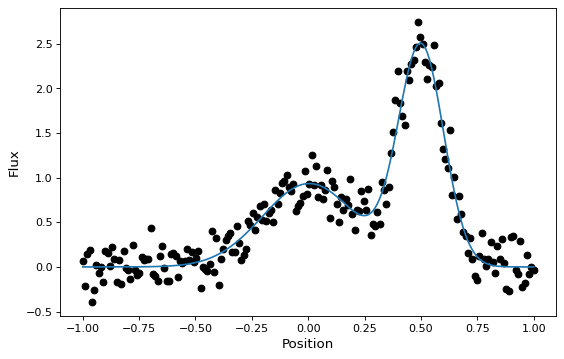Astropy模型还支持通过函数进行卷积 convolve_models ，返回一个复合模型。

import numpy as np
import matplotlib.pyplot as plt
from astropy.modeling import models
from astropy.convolution import convolve_models

g1 = models.Gaussian1D(1, -1, 1)
g2 = models.Gaussian1D(1, 1, 1)
g3 = convolve_models(g1, g2)

x = np.linspace(-3, 3, 50)
plt.plot(x, g1(x), 'k-')
plt.plot(x, g2(x), 'k-')
plt.plot(x, g3(x), 'k-')


(png _, svgpdf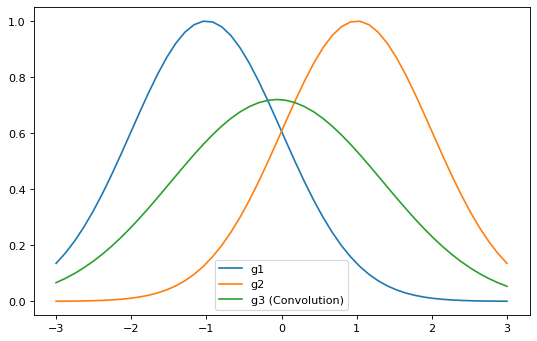## 全面的描述¶

### 一些术语¶

• 术语“模型”可以指模型 或者一个模型 实例 .

• 所有型号 astropy.modeling ，无论它是否代表 function ，A rotation 等等，在抽象中用一个模型表示 --特别是 Model --它封装了评估模型的例程、所需参数的列表以及有关模型的其他元数据。

• 按照典型的面向对象的说法，一个模型 实例 是在调用带有某些参数的模型类时创建的对象，在大多数情况下是模型参数的值。

模型类本身不能用于执行任何计算，因为大多数模型至少都有一个或多个参数，这些参数必须在对某些输入数据进行计算之前指定。但是，我们仍然可以从模型类的表示中获得一些有关它的信息。例如：：

>>> from astropy.modeling.models import Gaussian1D
>>> Gaussian1D
<class 'astropy.modeling.functional_models.Gaussian1D'>
Name: Gaussian1D
N_inputs: 1
N_outputs: 1
Fittable parameters: ('amplitude', 'mean', 'stddev')


然后我们可以创建一个模型 实例 通过传入三个参数的值：

>>> my_gaussian = Gaussian1D(amplitude=1.0, mean=0, stddev=0.2)
>>> my_gaussian
<Gaussian1D(amplitude=1.0, mean=0.0, stddev=0.2)>


我们现在有一个 实例 属于 Gaussian1D 它的所有参数（原则上还有其他细节，如拟合约束）都已填写，以便我们可以像函数一样使用它进行计算：

>>> my_gaussian(0.2)
0.6065306597126334


在许多情况下，本文档只提到“模型”，其中类/实例的区别要么无关紧要，要么与上下文无关。但在必要的时候会做出区分。

• A 复合模型 可以通过组合两个或多个现有模型实例来创建，这些实例可以是Astropy附带的模型， user defined models ，或其他复合模型——使用由一个或多个受支持的二进制运算符组成的Python表达式。模型类的组合已弃用，将在版本4.0中删除。

• 在某些地方 复合模型 可与互换使用 复合模型 . 然而，本文件使用了术语 复合模型 参考 only 对于使用管道操作符从两个或多个模型的功能组合创建的复合模型的情况 | 如下所述。此区别在本文档中一直使用，但理解其区别可能会有所帮助。

### 创建复合模型¶

>>> two_gaussians = Gaussian1D(1.1, 0.1, 0.2) + Gaussian1D(2.5, 0.5, 0.1)
>>> two_gaussians
<CompoundModel...(amplitude_0=1.1, mean_0=0.1, stddev_0=0.2, amplitude_1=2.5, mean_1=0.5, stddev_1=0.1)>


>>> two_gaussians(0.2)
0.9985190841886609


>>> print(two_gaussians)
Model: CompoundModel...
Inputs: ('x',)
Outputs: ('y',)
Model set size: 1
Expression:  + 
Components:
: <Gaussian1D(amplitude=1.1, mean=0.1, stddev=0.2)>

: <Gaussian1D(amplitude=2.5, mean=0.5, stddev=0.1)>
Parameters:
amplitude_0 mean_0 stddev_0 amplitude_1 mean_1 stddev_1
----------- ------ -------- ----------- ------ --------
1.1    0.1      0.2         2.5    0.5      0.1


>>> four_gaussians = two_gaussians + two_gaussians
>>> print(four_gaussians)
Model: CompoundModel...
Inputs: ('x',)
Outputs: ('y',)
Model set size: 1
Expression:  +  +  + 
Components:
: <Gaussian1D(amplitude=1.1, mean=0.1, stddev=0.2)>

: <Gaussian1D(amplitude=2.5, mean=0.5, stddev=0.1)>

: <Gaussian1D(amplitude=1.1, mean=0.1, stddev=0.2)>

: <Gaussian1D(amplitude=2.5, mean=0.5, stddev=0.1)>
Parameters:
amplitude_0 mean_0 stddev_0 amplitude_1 ... stddev_2 amplitude_3 mean_3 stddev_3
----------- ------ -------- ----------- ... -------- ----------- ------ --------
1.1    0.1      0.2         2.5 ...      0.2         2.5    0.5      0.1


### 模型组成¶

import numpy as np
import matplotlib.pyplot as plt
from astropy.modeling.models import RedshiftScaleFactor, Gaussian1D

x = np.linspace(0, 1.2, 100)
g0 = RedshiftScaleFactor(0) | Gaussian1D(1, 0.75, 0.1)

plt.figure(figsize=(8, 5))
plt.plot(x, g0(x), 'g--', label='$z=0$')

for z in (0.2, 0.4, 0.6):
g = RedshiftScaleFactor(z) | Gaussian1D(1, 0.75, 0.1)
plt.plot(x, g(x), color=plt.cm.OrRd(z),
label=f'$z={z}$')

plt.xlabel('Energy')
plt.ylabel('Flux')
plt.legend()


(png _, svgpdf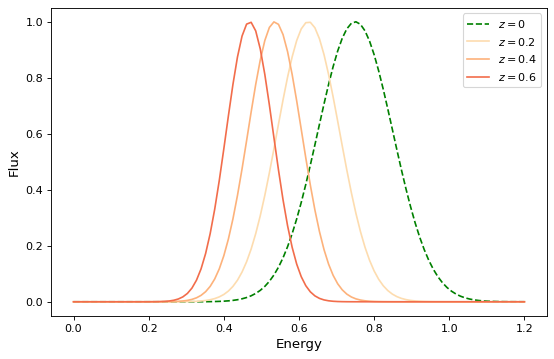import numpy as np
import matplotlib.pyplot as plt
from astropy.modeling.models import RedshiftScaleFactor, Gaussian1D, Scale

x = np.linspace(1000, 5000, 1000)
g0 = Gaussian1D(1, 2000, 200)  # No redshift is same as redshift with z=0

plt.figure(figsize=(8, 5))
plt.plot(x, g0(x), 'g--', label='$z=0$')

for z in (0.2, 0.4, 0.6):
rs = RedshiftScaleFactor(z).inverse  # Redshift in wavelength space
sc = Scale(1. / (1 + z))  # Rescale the flux to conserve energy
g = rs | g0 | sc
plt.plot(x, g(x), color=plt.cm.OrRd(z),
label=f'$z={z}$')

plt.xlabel('Wavelength')
plt.ylabel('Flux')
plt.legend()


(png _, svgpdf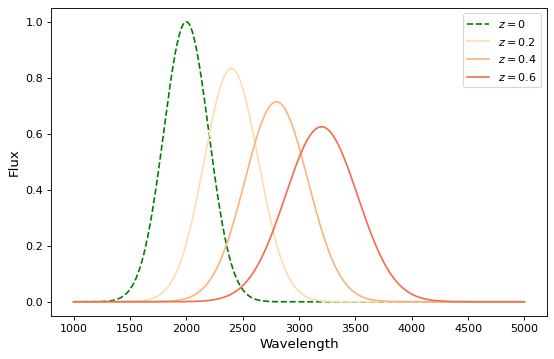import numpy as np
import matplotlib.pyplot as plt
from astropy.modeling.models import Rotation2D, Gaussian2D

x, y = np.mgrid[-1:1:0.01, -1:1:0.01]

plt.figure(figsize=(8, 2.5))

for idx, theta in enumerate((0, 45, 90)):
g = Rotation2D(theta) | Gaussian2D(1, 0, 0, 0.1, 0.3)
plt.subplot(1, 3, idx + 1)
plt.imshow(g(x, y), origin='lower')
plt.xticks([])
plt.yticks([])
plt.title(f'Rotated ${theta}^\circ$')


(png _, svgpdf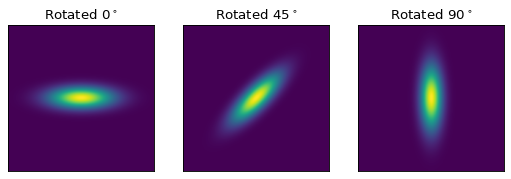>>> from astropy.modeling.models import Rotation2D
>>> Rotation2D() | Gaussian1D()
Traceback (most recent call last):
...
ModelDefinitionError: Unsupported operands for |: Rotation2D (n_inputs=2, n_outputs=2) and Gaussian1D (n_inputs=1, n_outputs=1); n_outputs for the left-hand model must match n_inputs for the right-hand model.


### 模型串联¶

>>> from astropy.modeling.models import Scale
>>> separate_scales = Scale(factor=1.2) & Scale(factor=3.4)
>>> separate_scales(1, 2)
(1.2, 6.8)


>>> scale_and_rotate = ((Scale(factor=1.2) & Scale(factor=3.4)) |
...                     Rotation2D(90))
>>> scale_and_rotate.n_inputs
2
>>> scale_and_rotate.n_outputs
2
>>> scale_and_rotate(1, 2)
(-6.8, 1.2)


>>> from numpy import allclose
>>> from astropy.modeling.models import AffineTransformation2D
>>> affine = AffineTransformation2D(matrix=[[0, -3.4], [1.2, 0]])
>>> # May be small numerical differences due to different implementations
>>> allclose(scale_and_rotate(1, 2), affine(1, 2))
True


## 其他主题¶

### 型号名称¶

>>> two_gaussians.name = "TwoGaussians"
>>> print(two_gaussians)
Model: CompoundModel...
Name: TwoGaussians
Inputs: ('x',)
Outputs: ('y',)
Model set size: 1
Expression:  + 
Components:
: <Gaussian1D(amplitude=1.1, mean=0.1, stddev=0.2)>

: <Gaussian1D(amplitude=2.5, mean=0.5, stddev=0.1)>
Parameters:
amplitude_0 mean_0 stddev_0 amplitude_1 mean_1 stddev_1
----------- ------ -------- ----------- ------ --------
1.1    0.1      0.2         2.5    0.5      0.1


### 索引和切片¶

>>> from astropy.modeling.models import Const1D
>>> A = Const1D(1.1, name='A')
>>> B = Const1D(2.1, name='B')
>>> C = Const1D(3.1, name='C')
>>> M = A + B * C
>>> print(M)
Model: CompoundModel...
Inputs: ('x',)
Outputs: ('y',)
Model set size: 1
Expression:  +  * 
Components:
: <Const1D(amplitude=1.1, name='A')>

: <Const1D(amplitude=2.1, name='B')>

: <Const1D(amplitude=3.1, name='C')>
Parameters:
amplitude_0 amplitude_1 amplitude_2
----------- ----------- -----------
1.1         2.1         3.1


>>> M
<Const1D(amplitude=2.1, name='B')>


>>> print(M[1:])
Model: CompoundModel
Inputs: ('x',)
Outputs: ('y',)
Model set size: 1
Expression:  * 
Components:
: <Const1D(amplitude=2.1, name='B')>

: <Const1D(amplitude=3.1, name='C')>
Parameters:
amplitude_0 amplitude_1
----------- -----------
2.1         3.1


m = m1 * m2 + m3


m1 * m2


m = m1 + m2 + m3 + m4


((m1 + m2) + m3) + m4


m = m1 + (m2 + m3) + m4. In this case m[1:3] will work.


>>> M[1:](0)
6.51


>>> print(M['B'])
Model: Const1D
Name: B
Inputs: ('x',)
Outputs: ('y',)
Model set size: 1
Parameters:
amplitude
---------
2.1


>>> print(M['B':'C'])
Model: CompoundModel...
Inputs: ('x',)
Outputs: ('y',)
Model set size: 1
Expression:  * 
Components:
: <Const1D(amplitude=2.1, name='B')>

: <Const1D(amplitude=3.1, name='C')>
Parameters:
amplitude_0 amplitude_1
----------- -----------
2.1         3.1


### 参数¶

>>> Gaussian1D.param_names
('amplitude', 'mean', 'stddev')
>>> (Gaussian1D() + Gaussian1D()).param_names
('amplitude_0', 'mean_0', 'stddev_0', 'amplitude_1', 'mean_1', 'stddev_1')


>>> from astropy.modeling.models import RedshiftScaleFactor
>>> (RedshiftScaleFactor() | (Gaussian1D() + Gaussian1D())).param_names
('z_0', 'amplitude_1', 'mean_1', 'stddev_1', 'amplitude_2', 'mean_2',
'stddev_2')


>>> a = Gaussian1D(1, 0, 0.2, name='A')
>>> b = Gaussian1D(2.5, 0.5, 0.1, name='B')
>>> m = a + b
>>> m.amplitude_0
Parameter('amplitude', value=1.0)


>>> m['A'].amplitude
Parameter('amplitude', value=1.0)


>>> m.amplitude_0 = 42
>>> m['A'].amplitude
Parameter('amplitude', value=42.0)
>>> m['A'].amplitude = 99
>>> m.amplitude_0
Parameter('amplitude', value=99.0)


>>> a.amplitude
Parameter('amplitude', value=99.0)


### 高级映射¶

Mapping models do not (currently) take any parameters, nor do they perform any numeric operation. They are for use solely with the concatenation (&) and composition (|) operators, and can be used to control how the inputs and outputs of models are ordered, and how outputs from one model are mapped to inputs of another model in a composition.

>>> m = (Scale(1.2) & Scale(3.4)) | Rotation2D(90)


>>> from astropy.modeling.models import Identity
>>> m = Scale(1.2) & Identity(1)
>>> m(1, 2)
(1.2, 2.0)


>>> m = Scale(1.2) & Identity(2)
>>> m(1, 2, 3)
(1.2, 2.0, 3.0)


（当然，最后一个例子也可以写出来 Scale(1.2) & Identity(1) & Identity(1)

>>> from astropy.modeling.models import Mapping
>>> m = Mapping((0,))
>>> m(1.0)
1.0


>>> m = Mapping((1, 0))
>>> m(1.0, 2.0)
(2.0, 1.0)

>>> m = Mapping((1, 0, 2))
>>> m(1.0, 2.0, 3.0)
(2.0, 1.0, 3.0)


>>> m = Mapping((1,))
>>> m(1.0, 2.0)
2.0

>>> m = Mapping((0, 2))
>>> m(1.0, 2.0, 3.0)
(1.0, 3.0)


>>> m = Mapping((0, 0))
>>> m(1.0)
(1.0, 1.0)

>>> m = Mapping((0, 1, 1, 2))
>>> m(1.0, 2.0, 3.0)
(1.0, 2.0, 2.0, 3.0)


>>> from astropy.modeling.models import Polynomial1D as Poly1D
>>> from astropy.modeling.models import Polynomial2D as Poly2D
>>> m = ((Poly1D(3, c0=1, c3=1) & Identity(1) & Poly1D(2, c2=1)) |
...      Mapping((0, 2, 1)) |
...      (Poly2D(4, c0_0=1, c1_1=1, c2_2=2) & Gaussian1D(1, 0, 4)))
...
>>> m(2, 3, 4)
(41617.0, 0.7548396019890073)


### 模型简化¶

：：
>>> order1_transform = fix_inputs(general_transform, {'order': 1})


### 替换子模型¶

replace_submodel() 通过将具有匹配名称的子模型替换为另一个子模型来创建新模型。新旧子模型的输入和输出数量应匹配。:

>>> from astropy.modeling import models
>>> shift = models.Shift(-1) & models.Shift(-1)
>>> scale = models.Scale(2) & models.Scale(3)
>>> scale.name = "Scale"
>>> model = shift | scale
>>> model(2, 1)
(2.0, 0.0)
>>> new_model = model.replace_submodel('Scale', models.Rotation2D(90, name='Rotation'))
>>> new_model(2, 1)
(6.12e-17, 1.0)
`